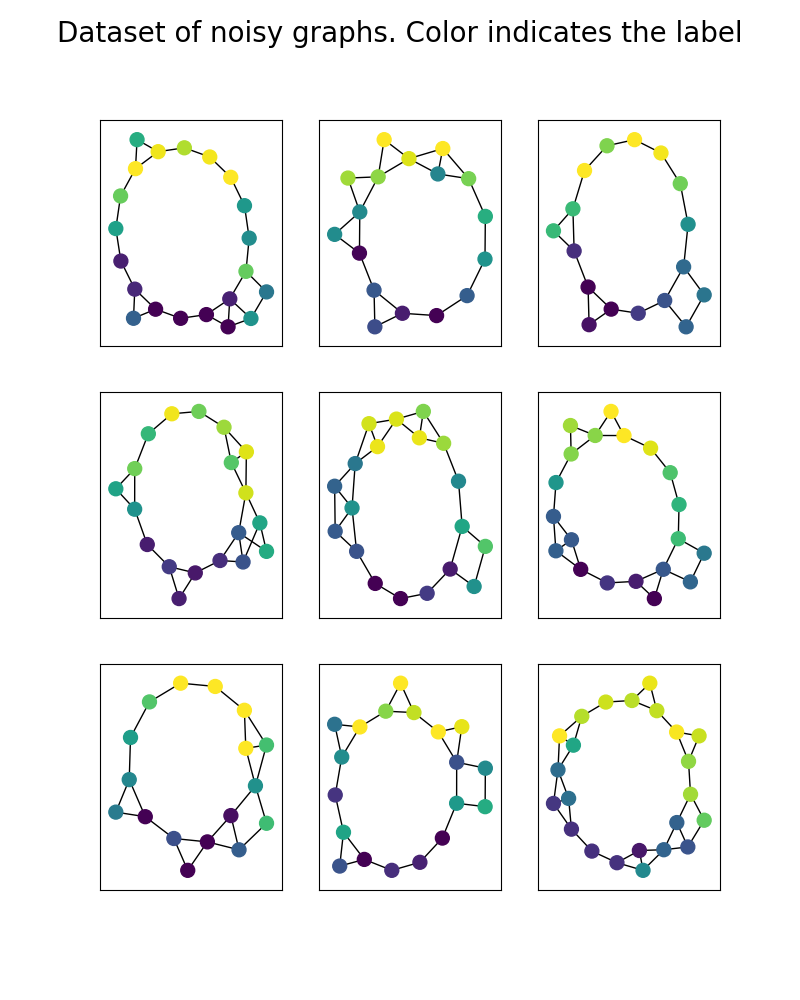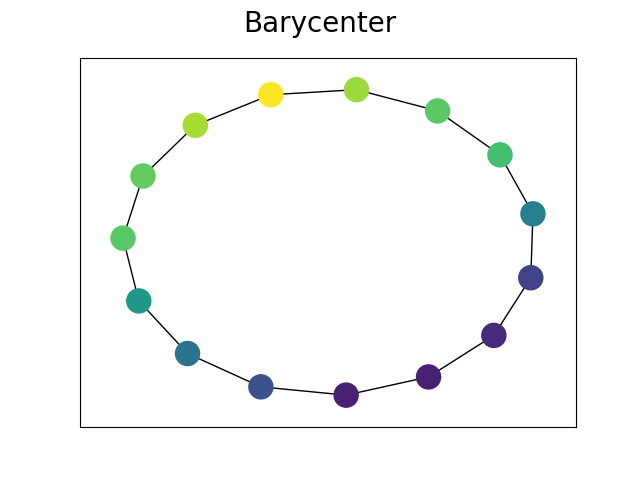# Plot graphs barycenter using FGW

This example illustrates the computation barycenter of labeled graphs using FGW .

Requires networkx >=2

 Vayer Titouan, Chapel Laetitia, Flamary Rémi, Tavenard Romain and Courty Nicolas “Optimal Transport for structured data with application on graphs” International Conference on Machine Learning (ICML). 2019.

```# Author: Titouan Vayer <titouan.vayer@irisa.fr>
#
```
```import numpy as np
import matplotlib.pyplot as plt
import networkx as nx
import math
from scipy.sparse.csgraph import shortest_path
import matplotlib.colors as mcol
from matplotlib import cm
from ot.gromov import fgw_barycenters
```
```def find_thresh(C, inf=0.5, sup=3, step=10):
""" Trick to find the adequate thresholds from where value of the C matrix are considered close enough to say that nodes are connected
Tthe threshold is found by a linesearch between values "inf" and "sup" with "step" thresholds tested.
The optimal threshold is the one which minimizes the reconstruction error between the shortest_path matrix coming from the thresholded adjency matrix
and the original matrix.
Parameters
----------
C : ndarray, shape (n_nodes,n_nodes)
The structure matrix to threshold
inf : float
The beginning of the linesearch
sup : float
The end of the linesearch
step : integer
Number of thresholds tested
"""
dist = []
search = np.linspace(inf, sup, step)
for thresh in search:
SC = shortest_path(Cprime, method='D')
SC[SC == float('inf')] = 100
dist.append(np.linalg.norm(SC - C))
return search[np.argmin(dist)], dist

""" Thresholds the structure matrix in order to compute an adjency matrix.
All values between threshinf and threshsup are considered representing connected nodes and set to 1. Else are set to 0
Parameters
----------
C : ndarray, shape (n_nodes,n_nodes)
The structure matrix to threshold
threshinf : float
The minimum value of distance from which the new value is set to 1
threshsup : float
The maximum value of distance from which the new value is set to 1
Returns
-------
C : ndarray, shape (n_nodes,n_nodes)
The threshold matrix. Each element is in {0,1}
"""
H = np.zeros_like(C)
np.fill_diagonal(H, np.diagonal(C))
C = C - H
C = np.minimum(np.maximum(C, threshinf), threshsup)
C[C == threshsup] = 0
C[C != 0] = 1

return C

def build_noisy_circular_graph(N=20, mu=0, sigma=0.3, with_noise=False, structure_noise=False, p=None):
""" Create a noisy circular graph
"""
g = nx.Graph()
for i in range(N):
noise = float(np.random.normal(mu, sigma, 1))
if with_noise:
g.add_node(i, attr_name=math.sin((2 * i * math.pi / N)) + noise)
else:
g.add_node(i, attr_name=math.sin(2 * i * math.pi / N))
if structure_noise:
randomint = np.random.randint(0, p)
if randomint == 0:
if i <= N - 3:
if i == N - 2:
if i == N - 1:
noise = float(np.random.normal(mu, sigma, 1))
if with_noise:
g.add_node(N, attr_name=math.sin((2 * N * math.pi / N)) + noise)
else:
g.add_node(N, attr_name=math.sin(2 * N * math.pi / N))
return g

def graph_colors(nx_graph, vmin=0, vmax=7):
cnorm = mcol.Normalize(vmin=vmin, vmax=vmax)
cpick = cm.ScalarMappable(norm=cnorm, cmap='viridis')
cpick.set_array([])
val_map = {}
for k, v in nx.get_node_attributes(nx_graph, 'attr_name').items():
val_map[k] = cpick.to_rgba(v)
colors = []
for node in nx_graph.nodes():
colors.append(val_map[node])
return colors
```

## Generate data

We build a dataset of noisy circular graphs. Noise is added on the structures by random connections and on the features by gaussian noise.

```np.random.seed(30)
X0 = []
for k in range(9):
X0.append(build_noisy_circular_graph(np.random.randint(15, 25), with_noise=True, structure_noise=True, p=3))
```

## Plot data

```plt.figure(figsize=(8, 10))
for i in range(len(X0)):
plt.subplot(3, 3, i + 1)
g = X0[i]
nx.draw(g, pos=pos, node_color=graph_colors(g, vmin=-1, vmax=1), with_labels=False, node_size=100)
plt.suptitle('Dataset of noisy graphs. Color indicates the label', fontsize=20)
plt.show()
```## Barycenter computation

Features distances are the euclidean distances

```Cs = [shortest_path(nx.adjacency_matrix(x)) for x in X0]
ps = [np.ones(len(x.nodes())) / len(x.nodes()) for x in X0]
Ys = [np.array([v for (k, v) in nx.get_node_attributes(x, 'attr_name').items()]).reshape(-1, 1) for x in X0]
lambdas = np.array([np.ones(len(Ys)) / len(Ys)]).ravel()
sizebary = 15  # we choose a barycenter with 15 nodes

A, C, log = fgw_barycenters(sizebary, Ys, Cs, ps, lambdas, alpha=0.95, log=True)
```

Out:

```/home/circleci/project/examples/gromov/plot_barycenter_fgw.py:164: FutureWarning: adjacency_matrix will return a scipy.sparse array instead of a matrix in Networkx 3.0.
Cs = [shortest_path(nx.adjacency_matrix(x)) for x in X0]
```

## Plot Barycenter

```bary = nx.from_numpy_matrix(sp_to_adjency(C, threshinf=0, threshsup=find_thresh(C, sup=100, step=100)))
for i, v in enumerate(A.ravel()):
```pos = nx.kamada_kawai_layout(bary)